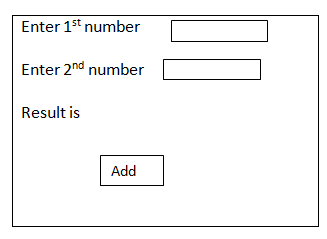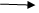Blog

## Razor syntax

21 September, 2018

## Performing same example with Razor syntax :-```@ layout = null;
Var num 1 = 0;
Var num 2 = 0;
Var Result = 0;
If (is post)
{
Num 1 = request [‘T1’].As Int [];
Num 2 = request [‘T2’].As Int [];
Result = num 1 + num2
}```

## Design :-In the first example we declare variable of int type and conversion is done by using Convert . to int 32 (); methodThis is completely in C # syntax. Here C# syntax with Razor syntax.In IInd Method we completely used Razor syntax that is Declaring the variable using keyword, and performing conversion using as int () method.
```Interested in mastering ASP.net Training?
1. Is Int ()compares the given data in integer type, return true. If the data belongs to integer type other vise return false.
2. Is Float ()compares the given data in F type, return true. If the data belongs to integer type other vise return false.
3. Is Decimal ()compares the given data in Decimal type, return true. If the data belongs to integer type other vise return false.
4. Is Date time ()compares the given data in Date time type, return true. If the data belongs to integer type other vise return false.
5. Is Bool ()compares the given data in Bool type, return true. If the data belongs to integer type other vise return false.